# Combinatorics problem

ms.math
Watch the image below. If we combine the two triangles we get different results. Triangles will be replaced with the number 3 (because triangles have three angles), the results obtained with the number as a geometric object angles. Connecting the two triangles is the mathematical operations of addition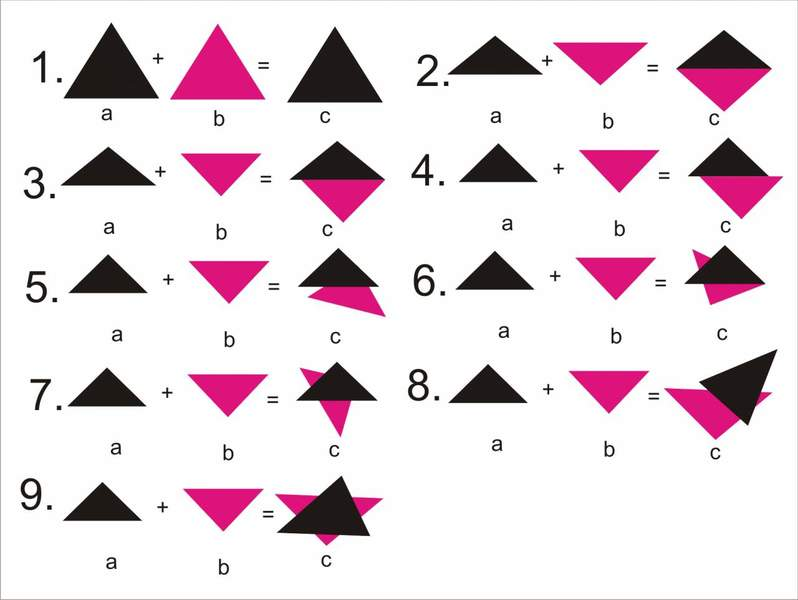a + b = c
1.3 +3 = 3
2.3 +3 = 4
3.3 +3 = 5
4.3 +3 = 6
5.3 +3 = 7
6.3 +3 = 8
7.3 +3 = 9
8.3 +3 = 10
9.3 +3 = 12
The current mathematics has the answer (4.3 +3 = 6), it is impossible for the other, the reality is that this may be true.
I'll show you a review of mathematics that solves problems, join ...

SteveL27

Yes, I see what you're doing. You're looking at all the different ways you can overlap two triangles to get various numbers of angles. I imagine there's some combinatorics going on. You could probably work out all the possibilities for overlapping any two polygons.

There's no contradiction between what you're doing and "current mathematics." Where are you going with this?

ms.math

. You could probably work out all the possibilities for overlapping any two polygons.
Yes. a(a>2)+b(b>2)=c -general form for all polygons
A different approach, a mathematical space that has two starting points (natural and realistic axiom - a natural geometric object and a real geometric object) in a mathematical space is monitored geometrical relationship between the object (function, numbers, logic equations, ..., are different names relations geometrical object).
Natural geometric object - along the natural (Figure AB)point the natural properties of longer, its beginning (A) and end (B) - the current point math is not defined.
Natural longer merge points. Each new geometric object should come from natural and long previous geometrical object (this is achieved by association of concepts and new concepts not as an axiom by the current math).
The following post is made to demonstrate the first evidence

Homework Helper
So you have redefined "addition". Nothing wrong with that as long as you understand that this is NOT "standard" addition and does not refute "standard" addition. And, in fact, it is pretty tame compared to general group theory.

Are you thinking there might be useful applications of this?

ms.math
So you have redefined "addition". Nothing wrong with that as long as you understand that this is NOT "standard" addition and does not refute "standard" addition. And, in fact, it is pretty tame compared to general group theory.

Are you thinking there might be useful applications of this?
When you look at this "addition", you will not understand, because you need to show much new to you realize
1.3 + (.0)3 = 3
2.3 +(.1)3 = 4
3.3 +(.2)3 = 5
4.3+3 = 6
5.33Rd1(6)d2(7)+3 = 7
6.33Rd1(6)d2(8)+3 = 8
7.33Rd1(6)d2(9)+3 = 9
8.33Rd1(6)d2(10)+3 = 10
9.33Rd1(6)d2(12)+3 = 12
if you look at the history of mathematics, you will see that the new things in math should take some time to have a practical application

--------------------------------------------
theorem - two (more) natural merge in the direction of longer AB

experiment (realization theorem)

we get the following geometric objects
1. final (n, in Figure 1.2.3.) Along
2. infinite (n, in Figure 4.) along a one-way infinite
--------------------------------------------------
asking - can you repeat back the title "ms.math" ( instead of combinatorics problem )

Last edited by a moderator:
ms.math
theorem - infinite point dc longer be replaced {(0), (0,1), ... (0,1,2,3,4,5,6,7,8,9), ...} circular and set position.
evidence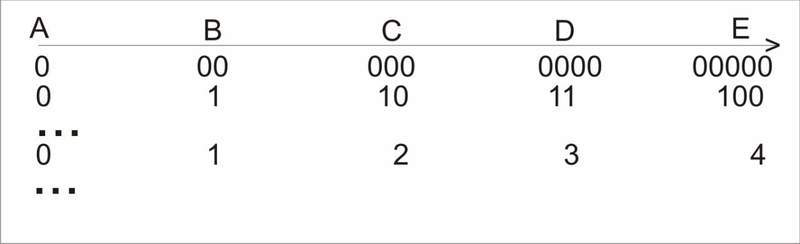We got a new geometric object - along the numerical

ms.math
Theorem - the length between points 0 and all points (separately) on the number the longer the new relationship
proof - look along the numerical
We got a set of natural numbers N = {0,1,2,3,4,5,6,7,8,9,10,11,12, ...},
example of the difference and the number of points on the number exceed:
Item 5 and No. 5 are two different things, point 5 is the point number 5 is the length between points 0 and 5 points along the numerical

ms.math
2.4 Mobile Number
Theorem-Natural numbers can be specified and other numerical
point other than the point numeric 0th
Proof - Value (length) numeric point (0) and numeric item (2)
the number 2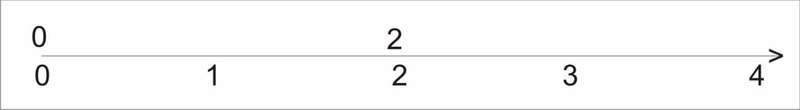Ratio (length) numeric point (1) and the numerical point of (3) is the number 2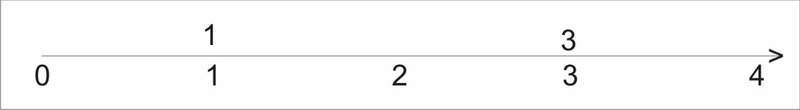Ratio (length) numeric point (2) and the numerical point of (4) is the number 2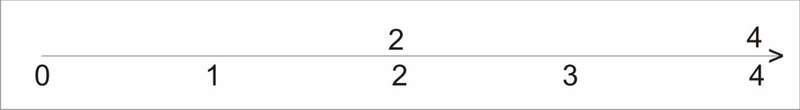...

ms.math
2.5 Gap numbers
Theorem- number and mobile number of no contact, ( number and mobile number without contact) and mobile number without con-
clock, ..., in numeric longer.
EVIDENCE - number 2 and mobile number 2 no contact, gets
a gap of 2 (.1.) 2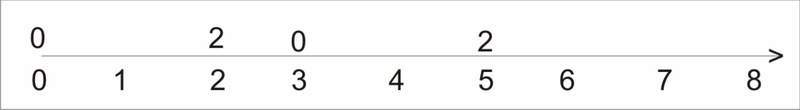number 2 and number mobile 2 no contact, getting the
2 (.2.) 2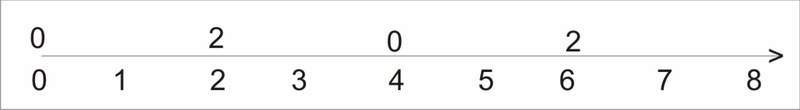number 2 and number mobile 2 no contact, getting the gap
2 (.3.) 2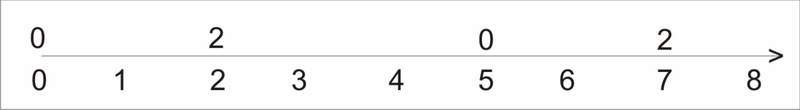...
(number 2 and mobile number 2 no contact) and mobile number 1
no contact, getting a gap of 2 (.1.) 2 (.1.) 1
...
Gap set of numbers GN={ a |(.bn.)cn | (a, bn, cn)$\in$ N, bn> 0}

Last edited:
ms.math
2.6 Moving of gap number
Theorem-gap numbers can be entered and the second numerical
point other than the point numeric 0
EVIDENCE-ratio (length) numeric point (0) and the numerical point of (4) is
gap 2 (.1.) 1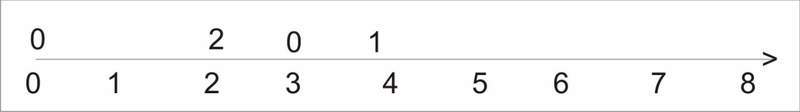Ratio (length) numeric point (1) and the numerical point of (5) is gap 2 (.1.) 1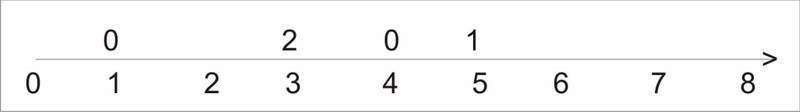Ratio (length) numeric point (2) and the numerical point of (6) is gap 2 (.1.) 1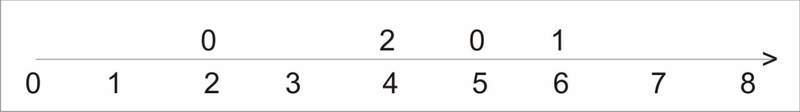...

ms.math
2.7 Points of number
Theorem - Number of numeric longer has a point, it could be the opposite
write.
EVIDENCE - Number 5 has a point: (.0), (.1), (.2), (.3) (.4) (.5). Opposite may
write: (.. 0), (​​.. 1), (​​2 ..) (.. 3), (​​4 ..) (.. 5).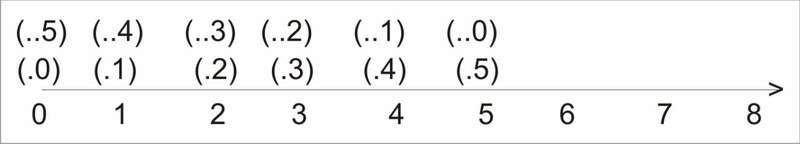Emptiness 2 (.3.) 1 has a point: (.0), (.1), (.2), (.3) (.4) (.5), (.6). can
Write the opposite: (.0), (​​.. 1), (.. 2), (​​3 ..) (.. 4), (​​5 ..) (.. 6)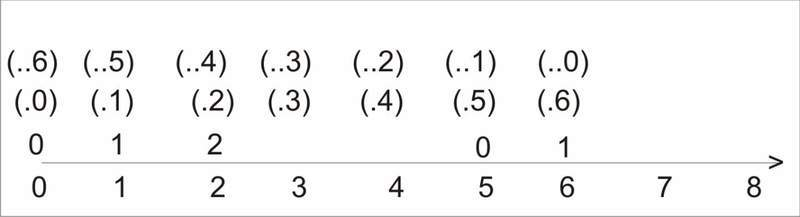ms.math
2.8 comparability of natural numbers

Theorem-Two (more) numbers are comparable to know
Who is the greater (equal, smaller), which is the point of (.. 0) away from the
numerical point of 0th
EVIDENCE - Two issues: 5> 3 (item number 5 (.5) away from the point
number 3 (.3)) 5 has a number of third 4 = 4 (item number 4 (.4) and the number of points
4 (.4) are equidistant) 4 is equal to 4 .2 <6 (item number 6 (.6) is
distance from point number 2 (.2) 2 less than 6 . ). (= {>, =, <}, a). (b.

Three issues: a). (b). (c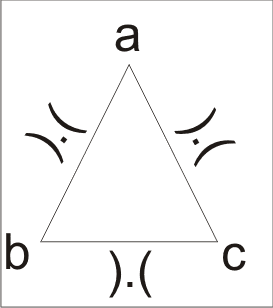...

Norwegian
Hi, msmath, are you serious about any of the above? I find it all completely incoherent. Would you mind explaining in complete sentences what is going on, and what your motivation for this is?

ms.math
Hi, msmath, are you serious about any of the above? I find it all completely incoherent. Would you mind explaining in complete sentences what is going on, and what your motivation for this is?
Every new thing emerges from the previous, my terms are associated
-------------
Theorem-number (number of gaps) and mobile number (mobile Gap
number) are in contact, the movable point number (mobile number gaps)
(.0) Varies according to the number of counts (number of gaps) and connect.
EVIDENCE - 3 + (.0) 3 = 3 or 3 + (.. 3) = 3.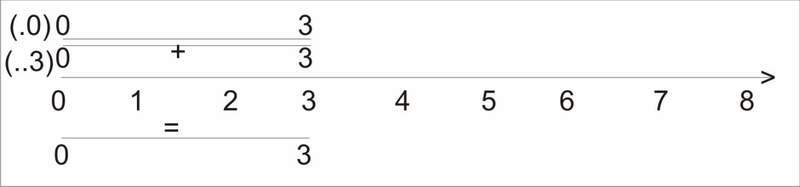3 + (.1) 3 = 4 or 3 + (.. 2) = 4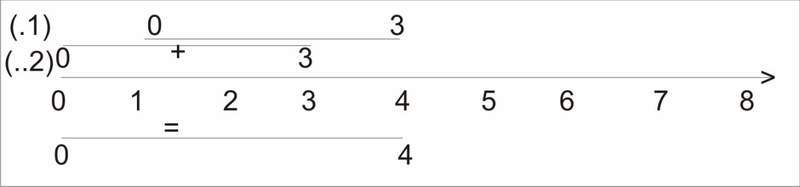3 + (.2) 3 = 5 or 3 + (.. 1) 3 = 5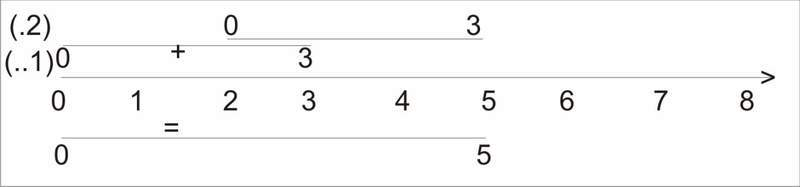3 + (.3) 3 = 6 or 3 + (.. 0) 3 = 6 or 3 +3 = 6